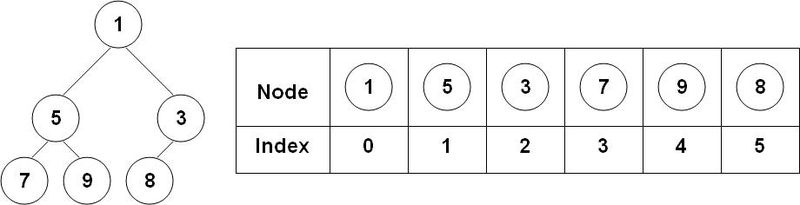## 堆（Heap）• 初始化堆：O(1)：在 Go 语言中，可以使用一个空切片或已经拥有元素的切片来初始化堆。
• 插入元素：O(log n)：插入元素时，先将元素添加到切片末尾，然后使用上浮操作（sift up）将新元素上移到合适的位置。在最坏情况下，需要执行 O(log n) 次上浮操作。
• 获取堆顶元素：O(1)：堆顶元素就是切片的第一个元素，获取它只需要 O(1) 的时间复杂度。
• 删除堆顶元素：O(log n)：删除堆顶元素时，先将切片的最后一个元素移到堆顶，然后使用下沉操作（sift down）将新的堆顶元素下沉到合适的位置。在最坏情况下，需要执行 O(log n) 次下沉操作。
• 修改堆中元素：O(log n)：修改堆中元素时，需要先找到元素在切片中的索引，然后根据新值和旧值的大小关系，分别执行上浮或下沉操作。在最坏情况下，需要执行 O(log n) 次上浮或下沉操作。

## 源码阅读

### interface

``````type Interface interface {
sort.Interface
Push(x interface{}) // add x as element Len()
Pop() interface{}   // remove and return element Len() - 1.
}``````

### down 和 up

heap操作中最重要的两个方法是`down``up`

down让一个节点下沉：

``````func down(h Interface, i0, n int) bool {
i := i0
for {
// j1为左子节点下标
j1 := 2*i + 1
if j1 >= n || j1 < 0 { // j1 < 0 after int overflow
break
}
j := j1 // left child
// j2为右子节点下标，j为最小的子节点的下标
if j2 := j1 + 1; j2 < n && !h.Less(j1, j2) {
j = j2 // = 2*i + 2  // right child
}
// 如果最小的子节点不小于父节点，则已经满足最小堆条件，退出
if !h.Less(j, i) {
break
}
// 如果最小的子节点小于父节点，则交换这两个节点
h.Swap(i, j)
// 让父节点继续下沉
i = j
}
return i > i0
}``````

up让一个节点上升：

``````func up(h Interface, j int) {
for {
// i为父节点下标
i := (j - 1) / 2 // parent
// 如果该节点不小于父节点，则满足最小堆条件，退出
if i == j || !h.Less(j, i) {
break
}
// 如果该节点小于父节点，则交换这两个节点
h.Swap(i, j)
// 让该节点继续上升
j = i
}
}``````

### Init

``````func Init(h Interface) {
n := h.Len()
// 最后一个节点下表为n-1，因此其父节点为(n-1-1)/2，即n/2-1
for i := n/2 - 1; i >= 0; i-- {
down(h, i, n)
}
}``````

### Push

`Push`的时候，先把新元素放置在结尾，然后让其上升。

``````func Push(h Interface, x interface{}) {
h.Push(x)
up(h, h.Len()-1)
}``````

### Pop

`Pop`的时候，先把根节点和最后一个节点交换位置，然后让新的根节点下降。注意这里down的第三个参数是n的值为h.Len() - 1，因此新的根节点下降的过程中，原先的根节点位于最后的位置，不受影响。

``````func Pop(h Interface) interface{} {
n := h.Len() - 1
h.Swap(0, n)
down(h, 0, n)
return h.Pop()
}``````

### Remove

`Remove`操作与`Pop`非常类似，先将需要移除的节点和最后一个节点交换位置，然后同时进行`down``up`操作。

``````func Remove(h Interface, i int) interface{} {
n := h.Len() - 1
if n != i {
h.Swap(i, n)
down(h, i, n)
up(h, i)
}
return h.Pop()
}``````

### Fix

``````func Fix(h Interface, i int) {
if !down(h, i, h.Len()) {
up(h, i)
}
}``````

## 使用 heap 包

### IntHeap 例子

``````import (
"container/heap"
"fmt"
)

type IntHeap []int

func (h IntHeap) Len() int           { return len(h) }
func (h IntHeap) Less(i, j int) bool { return h[i] < h[j] }
func (h IntHeap) Swap(i, j int)      { h[i], h[j] = h[j], h[i] }

func (h *IntHeap) Pop() interface{} {
old := *h
n := len(old)
// 将顶小堆元素与最后一个元素交换位置，在进行堆排序的结果
x := old[n-1]
*h = old[0 : n-1]
return x
}

// 实现 Push 方法，插入新元素
func (h *IntHeap) Push(x interface{}) {
*h = append(*h, x.(int))
}

func main() {
h := &IntHeap{2, 1, 5, 13,11, 4, 3, 7, 9, 8, 0}
heap.Init(h)                                // 将数组切片进行堆化
fmt.Println(*h)
fmt.Println(heap.Pop(h))                    // 调用 pop 0 返回移除的顶部最小元素
heap.Push(h, 6)                             // 添加一个元素进入堆中进行堆化
for len(*h) > 0 {
fmt.Printf("%d \n", heap.Pop(h))
}
}``````

### 优先队列例子

``````import (
"container/heap"
"fmt"
)

type Item struct {
value    string // 优先级队列中的数据，可以是任意类型，这里使用string
priority int    // 优先级队列中节点的优先级
index    int    // index是该节点在堆中的位置
}

// 优先级队列需要实现heap的interface
type PriorityQueue []*Item

// 绑定Len方法
func (pq PriorityQueue) Len() int {
return len(pq)
}

// 绑定Less方法，这里用的是小于号，生成的是小根堆
func (pq PriorityQueue) Less(i, j int) bool {
return pq[i].priority < pq[j].priority
}

// 绑定swap方法
func (pq PriorityQueue) Swap(i, j int) {
pq[i], pq[j] = pq[j], pq[i]
pq[i].index, pq[j].index = i, j
}

// 绑定put方法，将index置为-1是为了标识该数据已经出了优先级队列了
func (pq *PriorityQueue) Pop() interface{} {
old := *pq
n := len(old)
item := old[n-1]
*pq = old[0 : n-1]
item.index = -1
return item
}

// 绑定push方法
func (pq *PriorityQueue) Push(x interface{}) {
n := len(*pq)
item := x.(*Item)
item.index = n
*pq = append(*pq, item)
}

// 更新修改了优先级和值的item在优先级队列中的位置
func (pq *PriorityQueue) update(item *Item, value string, priority int) {
item.value = value
item.priority = priority
heap.Fix(pq, item.index)
}

func main() {
// 创建节点并设计他们的优先级
items := map[string]int{"二毛": 5, "张三": 3, "狗蛋": 9}
i := 0
pq := make(PriorityQueue, len(items)) // 创建优先级队列，并初始化
for k, v := range items {             // 将节点放到优先级队列中
pq[i] = &Item{
value:    k,
priority: v,
index:    i}
i++
}
heap.Init(&pq) // 初始化堆
item := &Item{ // 创建一个item
value:    "李四",
priority: 1,
}
heap.Push(&pq, item)           // 入优先级队列
pq.update(item, item.value, 6) // 更新item的优先级
for len(pq) > 0 {
item := heap.Pop(&pq).(*Item)
fmt.Printf("%.2d:%s index:%.2d\n", item.priority, item.value, item.index)
}
}``````
``````输出结果：
03:张三 index:-01
05:二毛 index:-01
06:李四 index:-01
09:狗蛋 index:-01``````

• 优先队列
• 定时器
• 求 TopK
• 求中位数

Responses